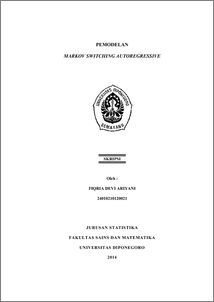# PEMODELAN MARKOV SWITCHING AUTOREGRESSIVE

ARIYANI, FIQRIA DEVI (2014) PEMODELAN MARKOV SWITCHING AUTOREGRESSIVE. Undergraduate thesis, FSM Undip.Preview
PDF
407Kb

## Abstract

Transition from depreciation to appreciation of exchange rate is one of regime switching that ignored by classic time series model, such as ARIMA, ARCH, or GARCH. Therefore, economic variables is modeled by Markov Switching Autoregressive (MSAR) which consider the regime switching. MLE is not applicable to parameters estimation because regime is an unobservable variable. So that filtering and smoothing process are applied to see the regime probabilities of observation. Using this model, transition probabilities and duration of the regime can be informed. In this case conducted exchange rate of Rupiah to US Dollar modeling with MSAR. The best model is MS(2)-AR(1) with transition probabilities from depreciation to appreciation is 0,052494 and appreciation to depreciation is 0,746716. Duration of the depreciation state is 19,04986 days and appreciation state is 1,339198 days. Keywords: regime switching, markov switching autoregressive, markov chain, transition probabilities, filtering and smoothing

Item Type: Thesis (Undergraduate) H Social Sciences > HA Statistics Faculty of Science and Mathematics > Department of Statistics 43613 Mr Hasbi Yasin 03 Sep 2014 15:50 03 Sep 2014 15:50

Repository Staff Only: item control page